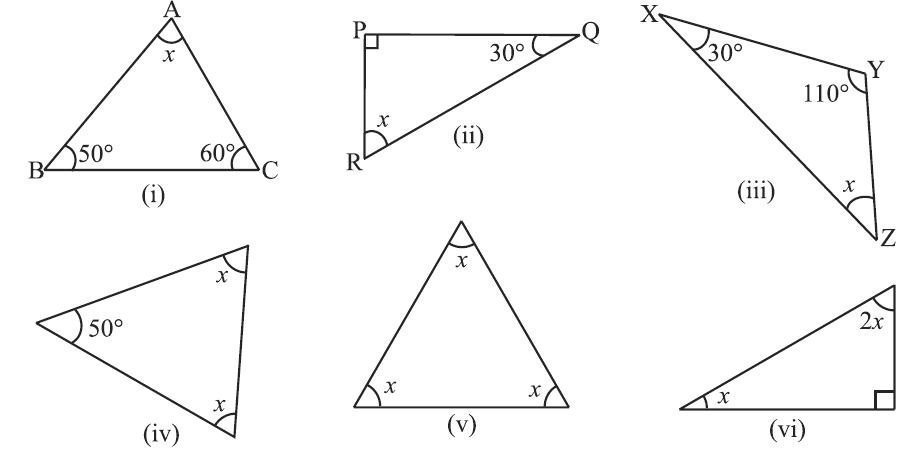In the verge of coronavirus pandemic, we are providing FREE access to our entire Online Curriculum to ensure Learning Doesn't STOP!

# Ex.6.3 Q1 The-Triangle-and-its-Properties Solution - NCERT Maths Class 7

Go back to  'Ex.6.3'

## Question

Find the value of the unknown $$x$$ in the following diagrams:Video Solution
Triangle & Its Properties
Ex 6.3 | Question 1

## Text Solution

What is known?

Measure of two interior angles of triangles.

What is unknown?

Value of one of the angles of the given triangles.

Reasoning:

We make use of the angle sum property of a triangle according to which the sum of the interior angles of a triangle is always equal to $$180^\circ.$$ If the given unknown interior angle of a triangle is $$x$$, then it can be obtained by subtracting sum of the other two angles from $$180^\circ$$

Steps:

(i) Sum of interior angles of a triangle $$= 180^\circ$$

\begin{align}∠A + ∠B + ∠C&=180^\circ\\ x+50^\circ+60^\circ&=180^\circ\\x=180^\circ-110^\circ&=70^\circ\\\end{align}

(ii) Sum of interior angles of a triangle $$= 180^\circ$$

\begin{align} \angle P+\angle Q+\angle R=180^\circ \\ 90^\circ +30^\circ +x=180^\circ \\ (\angle P=90^\circ \text{from figure}) \\ x=180^\circ -120^\circ =60^\circ \\ \end{align}

(iii) Sum of interior angles of a triangle $$= 180^\circ$$

\begin{align}∠X+ ∠Y+ ∠Z&=180^\circ\\ 30^\circ+110^\circ+x&=180^\circ\\ x=180^\circ-140^\circ&=40^\circ\\\end{align}

(iv) Sum of interior angles of a triangle $$= 180^\circ$$ (From step$$1$$)

\begin{align} x+x+50^\circ&=180^\circ\\2 x+50^\circ&=180^\circ\\2x=180^\circ&-50^\circ\\x\frac{130}{2}&=65^\circ\end{align}

(v) Sum of interior angles of a triangle $$= 180^\circ$$ (From step$$1$$)

\begin{align}x+x+x&=180^\circ\\ 3x&=180^\circ\\x\frac{180}{3}&=60^\circ\end{align}

(vi) Sum of interior angles of a triangle $$= 180^\circ$$ (From step $$1$$)

\begin{align} x+2x+90^\circ&=180^\circ\\3x+90^\circ&=180^\circ\\ 3x&=180^\circ-90^\circ\\x=\frac{90}{3}&=30{}^\circ \end{align}

Learn from the best math teachers and top your exams

• Live one on one classroom and doubt clearing
• Practice worksheets in and after class for conceptual clarity
• Personalized curriculum to keep up with school